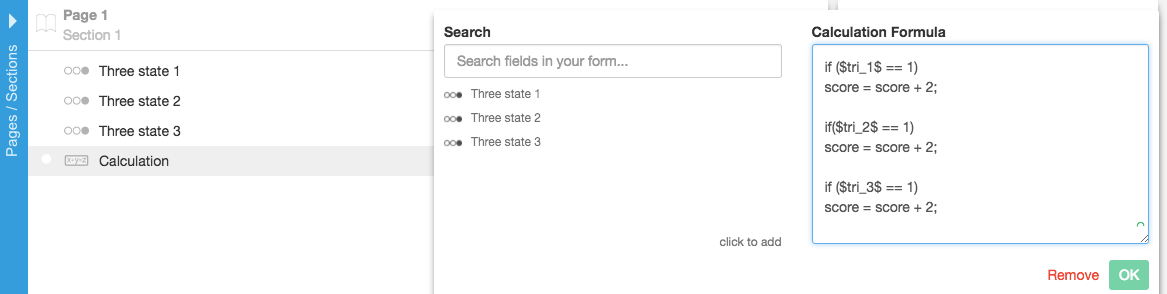Follow

Scoring - Yes/No/NA (Three State)

You can add scoring to your forms using Yes/No/NA selector fields, a calculation (computed label) field, and a little bit of Javascript. I will show you some basic code that you can tailor to your specific need in your forms. At this point, the article assumes that you have a basic understanding of the FastField software, how to add fields, save your forms, and view them on your device.

The code in this example is going to be a little bit more involved than the other scoring articles.

``````var score = 0;

if (\$tri_1\$ == 1)
score = score + 1;

if(\$tri_2\$ == 1)
score = score + 1;

if (\$tri_3\$ == 1)
score = score + 1;

return score;
``````

This is going to add all of the "Yes" selections in the form and display it in the computed label. If the user selects Yes, No, No, then the computed label will display "1". You can add whatever weight to the Yes selection that you would like. For example...

``````var score = 0;

if (\$tri_1\$ == 1)
score = score + 2;

if(\$tri_2\$ == 1)
score = score + 2;

if (\$tri_3\$ == 1)
score = score + 2;

return score;
``````

If you select Yes, Yes, No then the calculation (computed label) will display "4" because you've given the Yes a weight of 2 instead of 1.In the example below, we selected all Yes with a weight of 1. The total score equals 3Have more questions? Submit a request